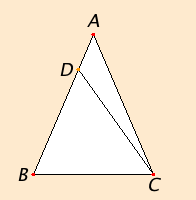# Proposition 6

If in a triangle two angles equal one another, then the sides opposite the equal angles also equal one another.

Let ABC be a triangle having the angle ABC equal to the angle ACB.I say that the side AB also equals the side AC.

C.N

If AB does not equal AC, then one of them is greater.

Let AB be greater. Cut off DB from AB the greater equal to AC the less, and join DC.

Since DB equals AC, and BC is common, therefore the two sides DB and BC equal the two sides AC and CB respectively, and the angle DBC equals the angle ACB. Therefore the base DC equals the base AB, and the triangle DBC equals the triangle ACB, the less equals the greater, which is absurd. Therefore AB is not unequal to AC, it therefore equals it.

Therefore if in a triangle two angles equal one another, then the sides opposite the equal angles also equal one another.

Q.E.D.

## Guide

#### Converses of propositions

This is the converse of (part of) the previous proposition I.5. Proposition I.6 says that if angle B equals angle C, then side AB equals side AC. Proposition I.5 says that if side AB equals side AC, then angle B equals angle C. In general, the converse of a proposition of the form “If P, then Q” is the proposition “If Q, then P.” When both a proposition and its converse are valid, Euclid tends to prove the converse soon after the proposition, a practice that has continued to this day.

A proposition and its converse are not logically equivalent. There are examples where “If P, then Q” is valid, but “If Q, then P” is not valid. An example from the Elements is proposition III.5 which states “If two circles cut one another, then they do not have the same center.” The converse would be “If two circles do not have the same center, then they cut one another” which is certainly not valid since if one circle lies entirely outside the other, then they don’t cut one another.

This is the first proof by contradiction, also called reductio ad absurdum, in the Elements. In this proof, in order to prove AB equals AC, Euclid assumes they are unequal and derives a contradiction, namely, that the triangle ACB equals a part of itself, triangle DBC, which contradicts C.N.5, the whole is greater than the part. The contradiction is that triangle ACB both equals and does not equal triangle DBC.

In general, to prove a statement of the form “P” with a proof by contradiction, begin with an assumption “not P” and derive some contradiction “Q and not Q,” and finally conclude “P.”

Euclid often uses proofs by contradiction, but he does not use them to conclude the existence of geometric objects. That is, he does not use them in constructions. But he does use them to show what has been constructed is correct.

In modern mathematics nonconstructive proofs by contradiction do occur. Famous examples are Brouwer’s fixed point theorems published in 1912. One of these states that any continuous transformation f of a circle (circular disk) to itself has a fixed point x, that is, a point such that f(x) = x. In his proof, he assumed that such a point did not exist and derived a contradiction. Although his proof is logically correct, he was not satisfied since the proof does not help in constructing a fixed point. Brouwer was an adherent of a philosophy of mathematics called intuitionism that holds, among other things, that mathematical objects have not been shown to exist until constructions have been given for them.

#### The law of trichotomy in practice

The proof uses the law of trichotomy for lines: “If AB does not equal AC, then one of them is greater.” There are three cases: AB < AC, AB = AC, or AB > AC. If the middle possibility is excluded, then only the two others remain, so one of the lines is greater. The law of trichotomy is not explicitly stated as a Common Notion, but it is the sort of property of magnitudes listed as Common Notions.

Proposition I.3 can be read as a construction to determine whether one line is less than, equal to, or greater than another. Using I.3, one line is laid along another, and it will fall short, fall equal, or extend beyond the other. For this proposition I.6, the construction simplifies since the two lines AB and AC already have one end in common.

The other part of the law of trichotomy is also used in the proof, the part that says only one of the three cases can occur: “... the triangle DBC equals the triangle ACB, the less equals the greater, which is absurd.” C.N.5, the whole is greater than the part, allows the conclusion that triangle DBC (the part) is less than triangle ACB (the whole). But the contradiction arises because only one of the two cases DBC = ACB and DBC < ACB can occur.

#### Use of Proposition 6

This proposition is not used in the proofs of any of the later propositions in Book I, but it is used in Books II, III, IV, VI, and XIII.